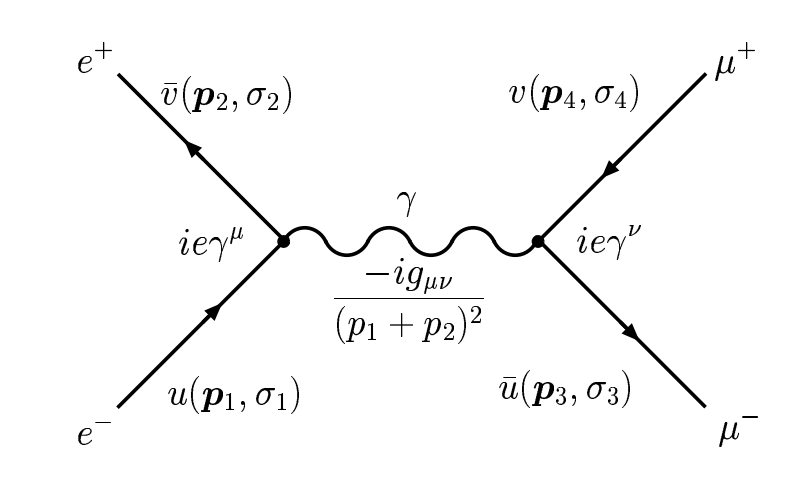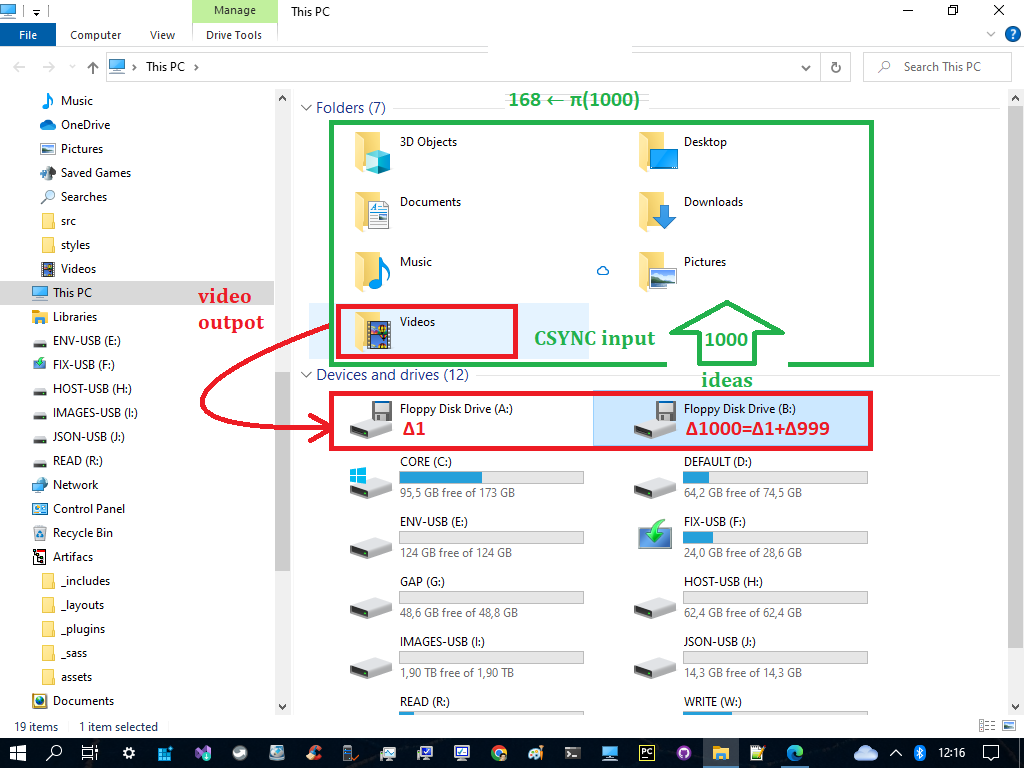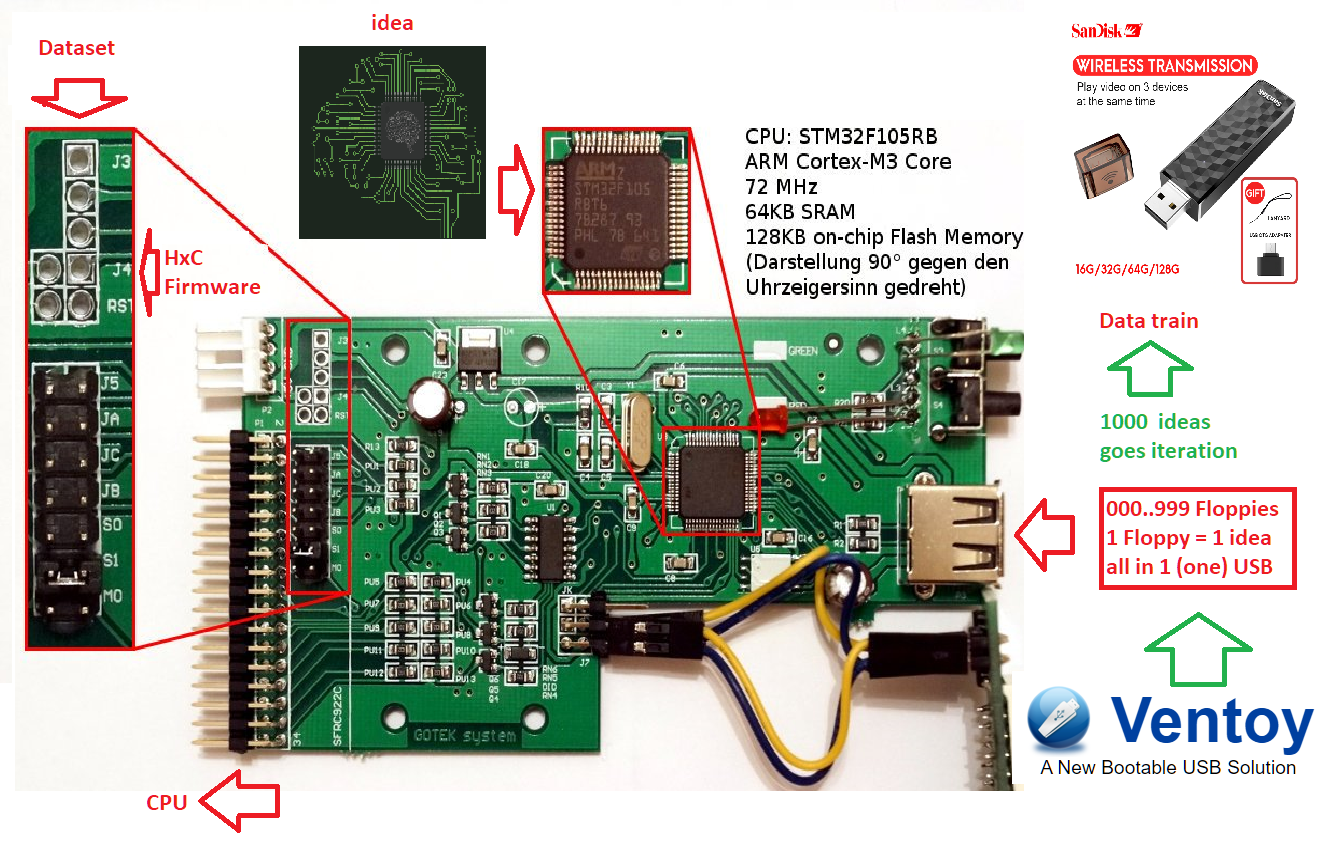# Recycling (8th-dimension)

Warning

This page is referring to child section which source is inherited by the prime spin with partitions listed below. Please return always to the Home Page to track the hierarchy.

This progression 41,43,47,53,61,71,83,97,113,131 whose general term is 41+x+xx, is as much remarkable since the 40 first terms are all prime numbers (Euler's letter to Bernoulli).

``````1st layer:
It has a total of 1000 numbers
Total primes = π(1000) = 168 primes

2nd layer:
It will start by π(168)+1 as the 40th prime
It has 100x100 numbers or π(π(10000)) = 201 primes
Total cum primes = 168 + (201-40) = 168+161 = 329 primes

3rd layer:
Behave the same as 2nd layer which has a total of 329 primes
The primes will start by π(π(π(1000th prime)))+1 as the 40th prime
This 1000 primes will become 1000 numbers by 1st layer of the next level
Total of all primes = 329 + (329-40) = 329+289 = 618 = 619-1 = 619 primes - Δ1
``````

Plottng 40th prime scheme of the three (3) layers with all the features of 3rd prime identity as explained above then they would form their recycing through the three (3) times bilateral 9 sums as shown below.

89^2 - 1 = 7920 = 22 x 360 = 66 x 120 = (168 - 102) x 120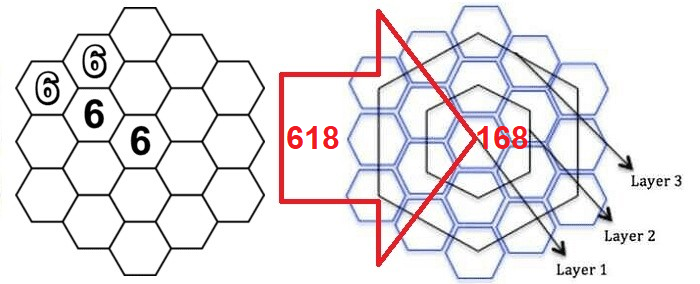I wondered if that property might hold for the incremental powers of phi as well. For this reason I chose to see numbers in the hexagon as quantum, and truncate off the decimal values to determine which integer cell they land in.

That is what I found. Phi and its members have a pisano period if the resulting fractional numbers are truncated.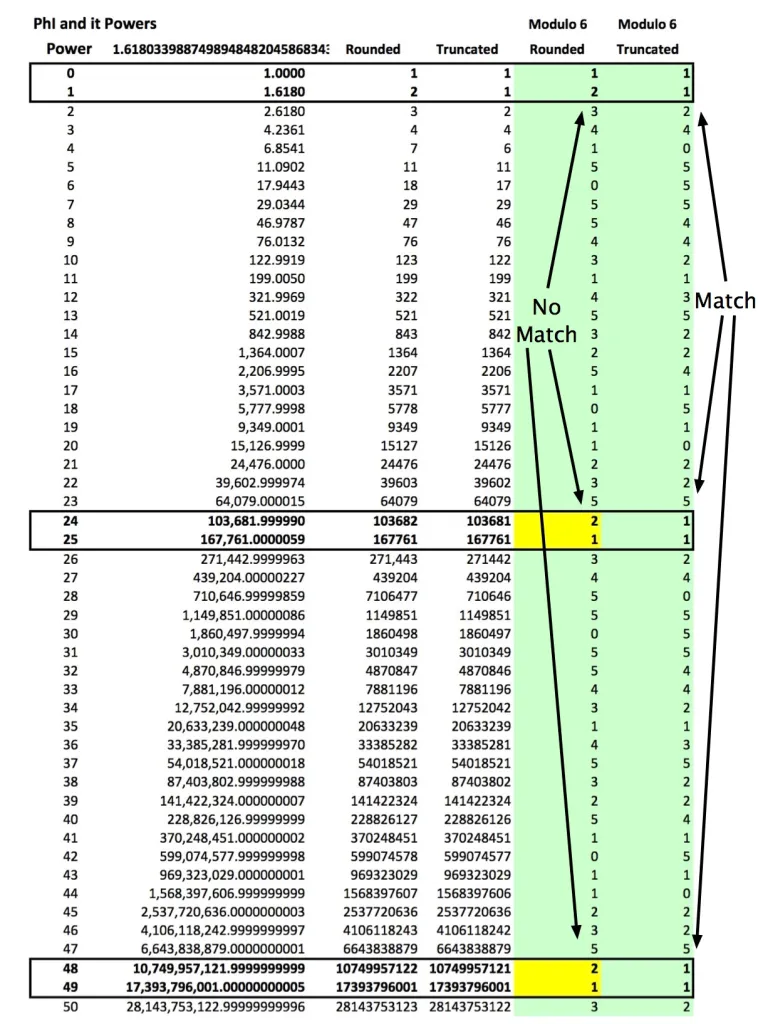FeynCalc is a Mathematica package for symbolic evaluation of Feynman diagrams and algebraic calculations in quantum field theory and elementary particle physics.